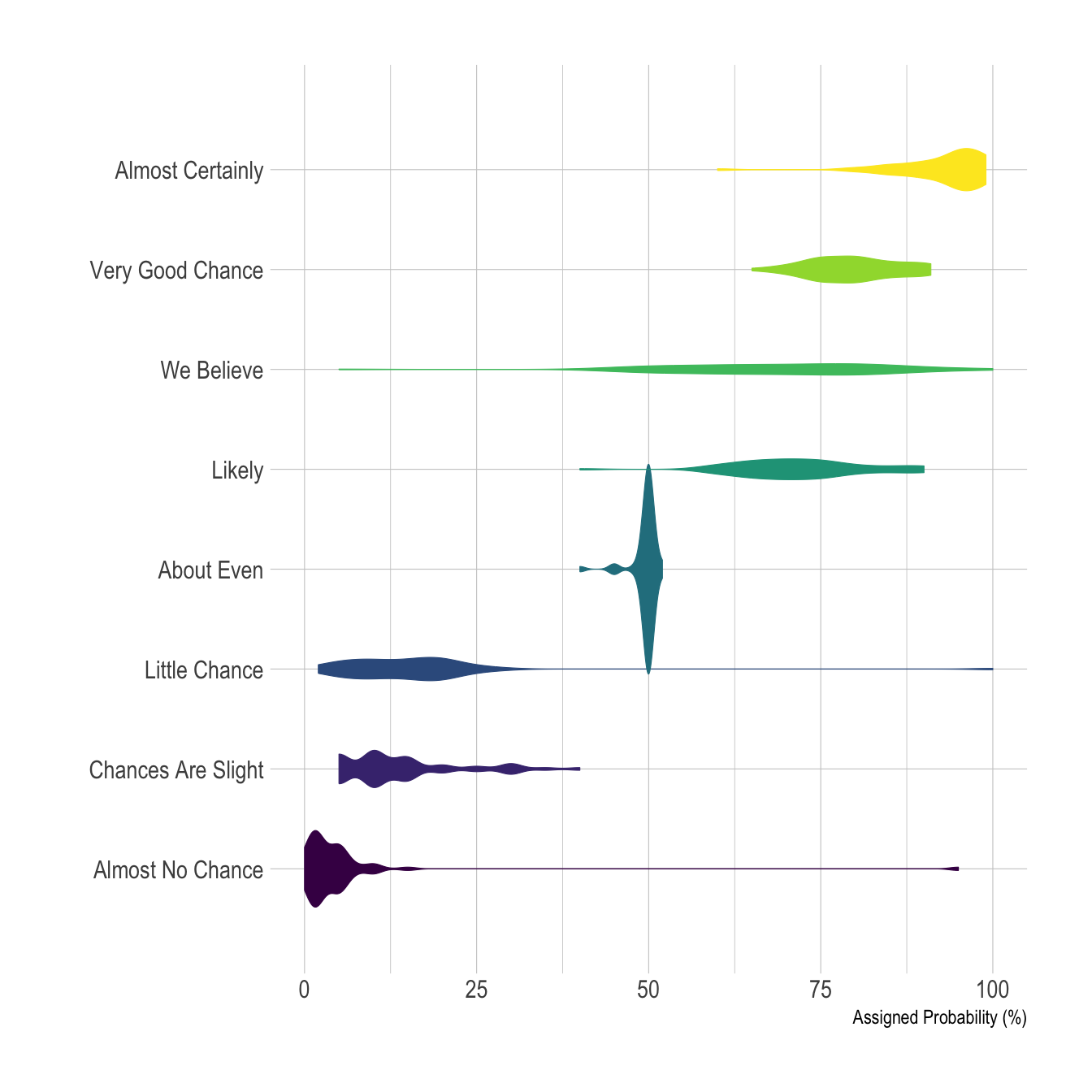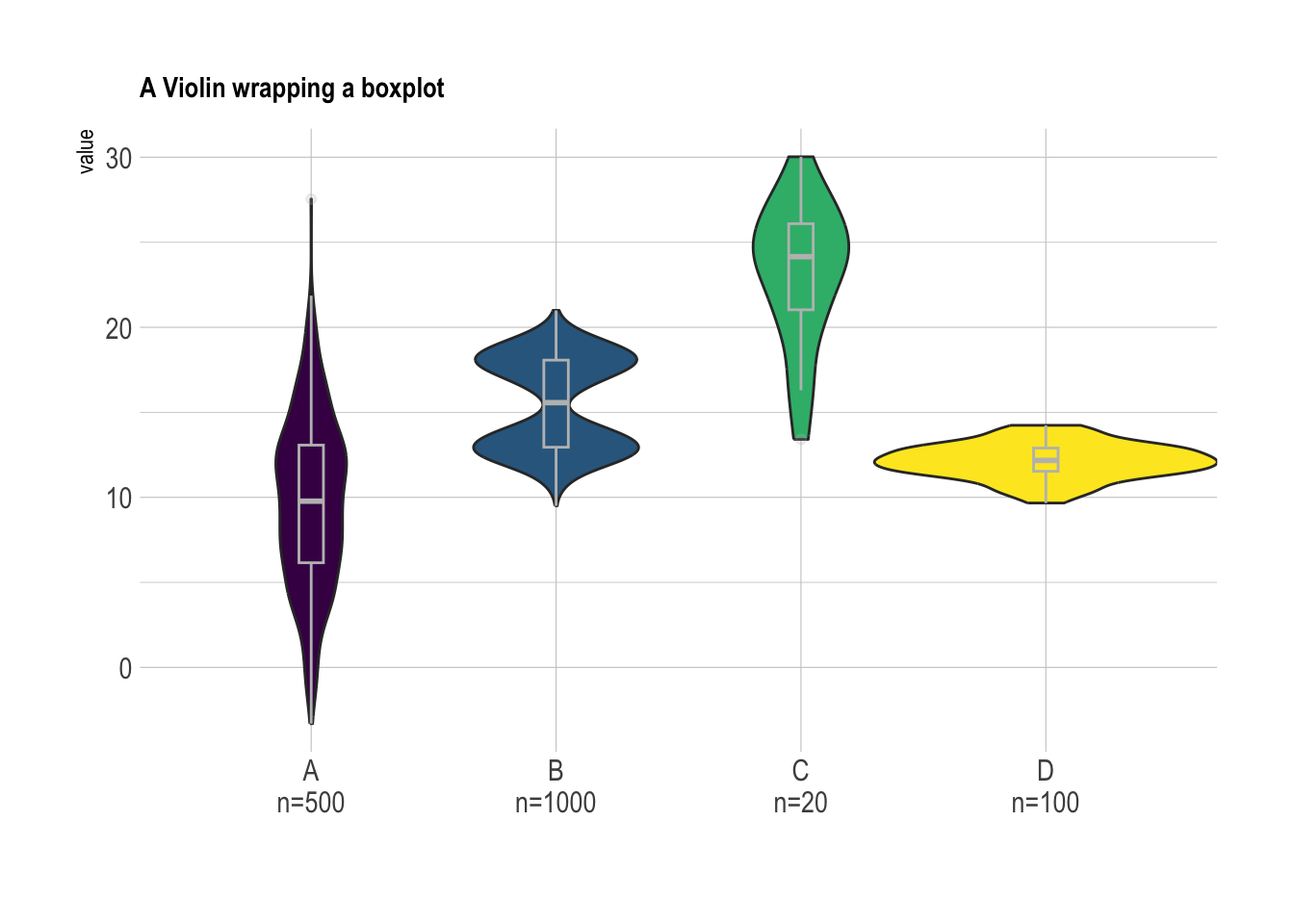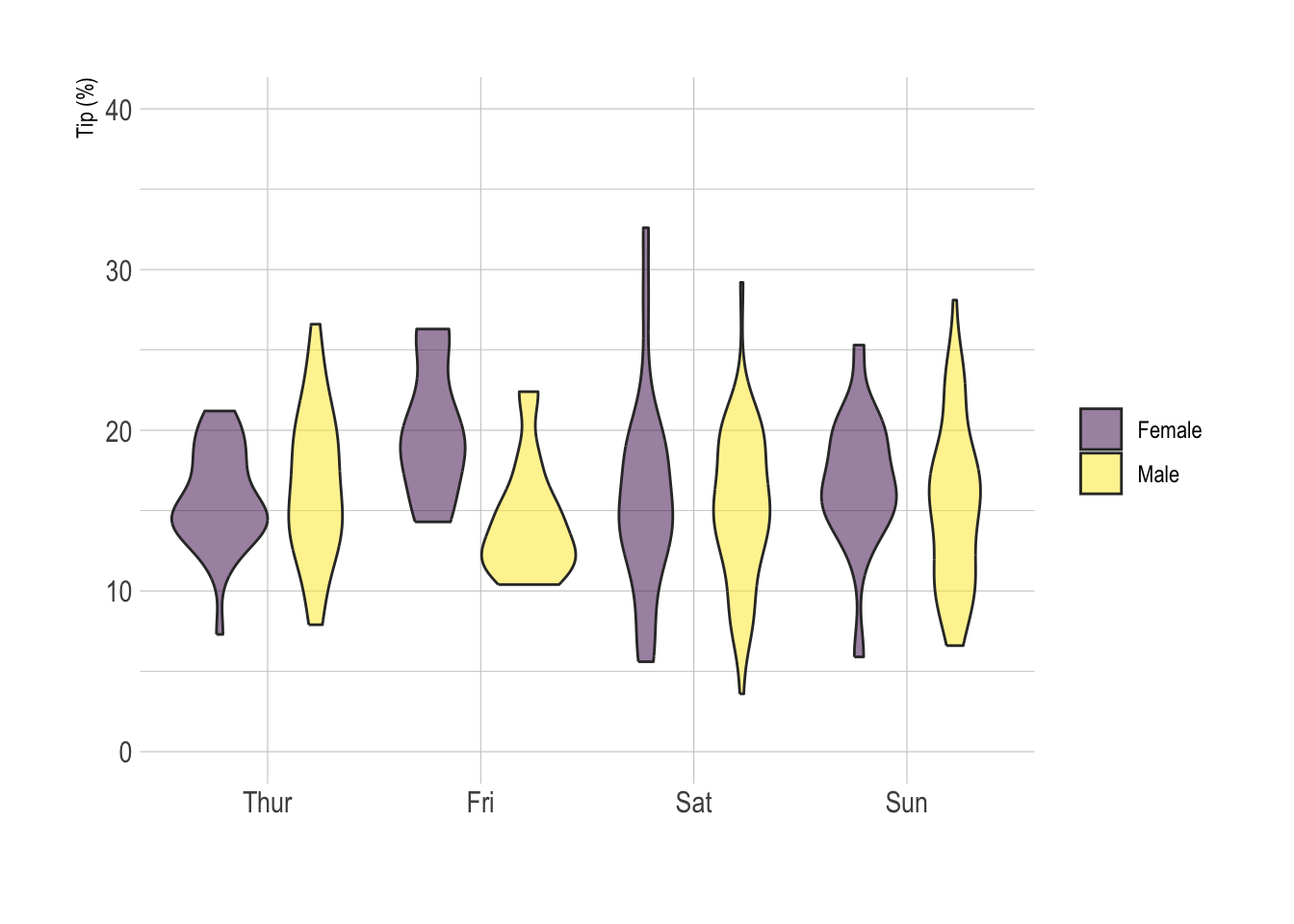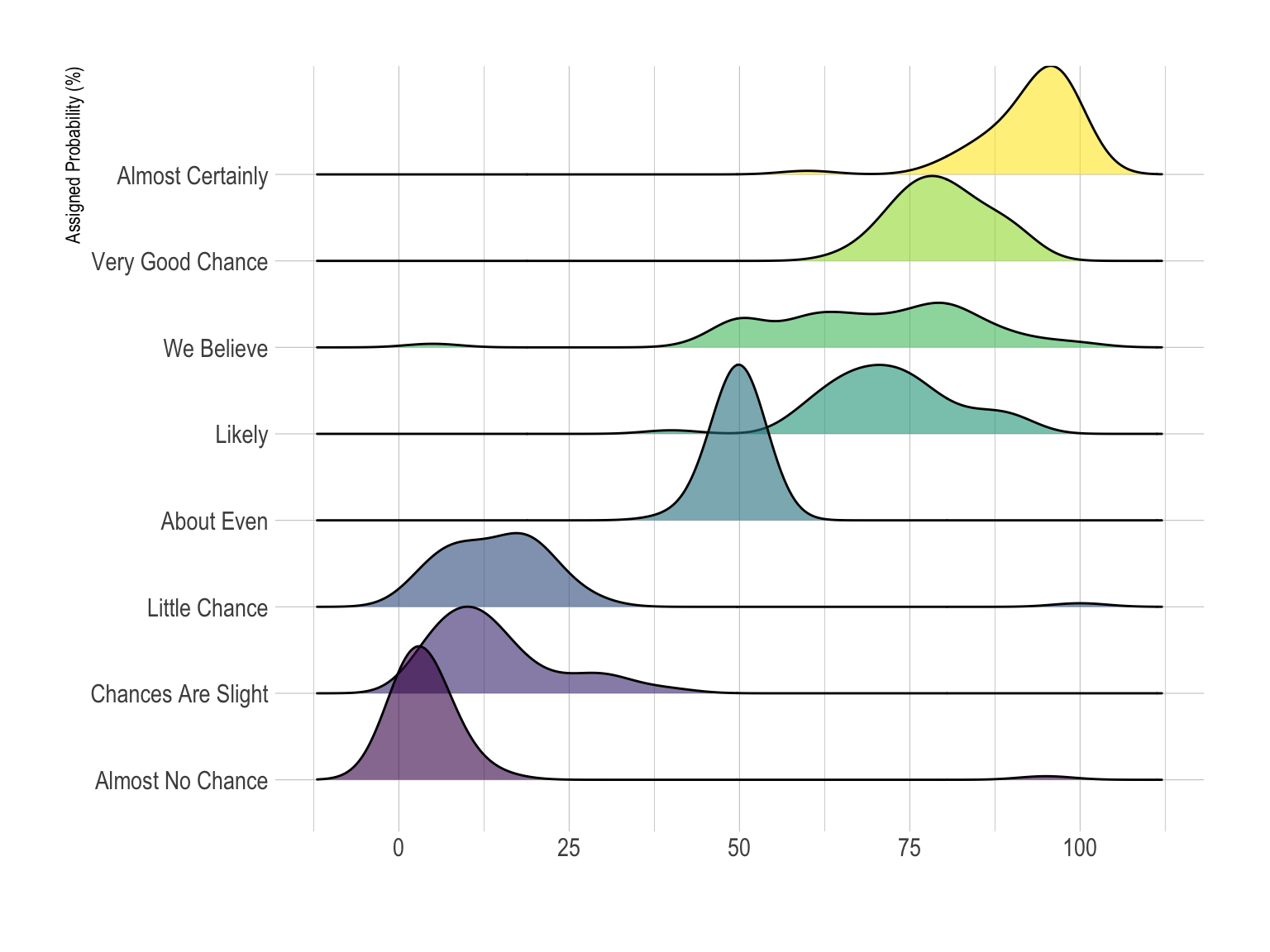# Definition

Violin plot allows to visualize the distribution of a numeric variable for one or several groups. Each ‘violin’ represents a group or a variable. The shape represents the density estimate of the variable: the more data points in a specific range, the larger the violin is for that range. It is really close to a boxplot, but allows a deeper understanding of the distribution.

Here is an example showing how people perceive probability. On the /r/samplesize thread of reddit, questions like What probability would you assign to the phrase “Highly likely” were asked. Answers between 0 and 100 were recorded, and here is the distribution for each question:

``````# Libraries
library(tidyverse)
library(hrbrthemes)
library(viridis)

data <- data %>%
gather(key="text", value="value") %>%
mutate(text = gsub("\\.", " ",text)) %>%
mutate(value = round(as.numeric(value),0)) %>%
filter(text %in% c("Almost Certainly","Very Good Chance","We Believe","Likely","About Even", "Little Chance", "Chances Are Slight", "Almost No Chance"))

# Plot
data %>%
mutate(text = fct_reorder(text, value)) %>%
ggplot( aes(x=text, y=value, fill=text, color=text)) +
geom_violin(width=2.1, size=0.2) +
scale_fill_viridis(discrete=TRUE) +
scale_color_viridis(discrete=TRUE) +
theme_ipsum() +
theme(
legend.position="none"
) +
coord_flip() +
xlab("") +
ylab("Assigned Probability (%)")``````Disclaimer: This idea originally comes from a publication of the CIA which resulted in this figure. Then, Zoni Nation cleaned the reddit dataset and built graphics with R.

# What for

Violin plot is a powerful data visualization technique since it allows to compare both the `ranking` of several groups and their `distribution`. Surprisingly, it is less used than boxplot, even if it provides more information in my opinion.

Violins are particularly adapted when the amount of data is huge and showing individual observations gets impossible. For small datasets, a boxplot with jitter is probably a better option since it really shows all the information.

# Variation

• Violin plot are made `vertically` most of the time. If you have long labels, building an `horizontal` version like above make the labels more readable.

• It is possible to display a boxplot in the violin: it allows to assess the median and quartiles in a glimpse. See the boxplot section for more info.

``````# create a dataset
data <- data.frame(
name=c( rep("A",500), rep("B",500), rep("B",500), rep("C",20), rep('D', 100)  ),
value=c( rnorm(500, 10, 5), rnorm(500, 13, 1), rnorm(500, 18, 1), rnorm(20, 25, 4), rnorm(100, 12, 1) )
)

# sample size
sample_size = data %>% group_by(name) %>% summarize(num=n())

# Plot
data %>%
left_join(sample_size) %>%
mutate(myaxis = paste0(name, "\n", "n=", num)) %>%
ggplot( aes(x=myaxis, y=value, fill=name)) +
geom_violin(width=1.4) +
geom_boxplot(width=0.1, color="grey", alpha=0.2) +
scale_fill_viridis(discrete = TRUE) +
theme_ipsum() +
theme(
legend.position="none",
plot.title = element_text(size=11)
) +
ggtitle("A Violin wrapping a boxplot") +
xlab("")``````• If your variable are grouped, you can build a grouped violin as you would do for a boxplot. Here is an example showing how much Male and Female tip depending on the day of the week.
``````# Load dataset from github
mutate(tip = round(tip/total_bill*100, 1))

# Grouped
data %>%
mutate(day = fct_reorder(day, tip)) %>%
mutate(day = factor(day, levels=c("Thur", "Fri", "Sat", "Sun"))) %>%
ggplot(aes(fill=sex, y=tip, x=day)) +
geom_violin(position="dodge", alpha=0.5, outlier.colour="transparent") +
scale_fill_viridis(discrete=T, name="") +
theme_ipsum()  +
xlab("") +
ylab("Tip (%)") +
ylim(0,40)``````# Common caveats

• If you compare groups with very different sample size, show it.
• Ordering groups by median value makes the chart more insightful.
• If you have just a few groups, you are probably interested by ridgeline charts. Here is a version for the probability perception story:
``````# Load dataset from github
data <- data %>%
gather(key="text", value="value") %>%
mutate(text = gsub("\\.", " ",text)) %>%
mutate(value = round(as.numeric(value),0)) %>%
filter(text %in% c("Almost Certainly","Very Good Chance","We Believe","Likely","About Even", "Little Chance", "Chances Are Slight", "Almost No Chance"))

library(ggridges)

data %>%
mutate(text = fct_reorder(text, value)) %>%
ggplot( aes(y=text, x=value,  fill=text)) +
geom_density_ridges(alpha=0.6, bandwidth=4) +
scale_fill_viridis(discrete=TRUE) +
scale_color_viridis(discrete=TRUE) +
theme_ipsum() +
theme(
legend.position="none",
panel.spacing = unit(0.1, "lines"),
strip.text.x = element_text(size = 8)
) +
xlab("") +
ylab("Assigned Probability (%)")``````# Related

The R and Python graph galleries are 2 websites providing hundreds of chart example, always providing the reproducible code. Click the button below to see how to build the chart you need with your favorite programing language.